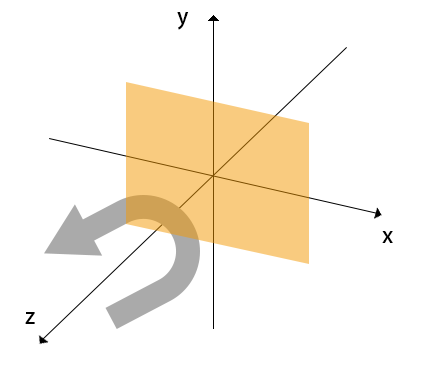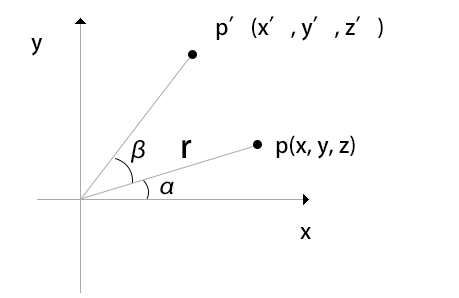WebGL图形变换：旋转矩阵 发布于：2023-02-26 20:45:33   原创发表   查看：45  讨论：0

1. 旋转轴（图形将围绕旋转轴旋转）
2. 旋转方向（方向：顺时针或逆时针）
3. 旋转角度（图形旋转经过的角度）x = r cos α
y = r sin α

x' = r cos (α + β)
y' = r sin (α + β)

x' = r (cos α cos β - sin α sin β)
y' = r (sin α cos β + cos α sin β)

x' = x cos β - y sin β
y' = y cos β + x sin β
z' = z

gl_Position.x = a_Position.x * u_CosB - a_Position.y * u_SinB;
gl_Position.y = a_Position.x * u_SinB + a_Position.y * u_CosB;
js：
var u_CosB = gl.getUniformLocation(gl.program, "u_CosB");
var u_SinB = gl.getUniformLocation(gl.program, "u_SinB");
gl.uniform1f(u_CosB );
gl.uniform1f(u_SinB );## 发表评论

•• 匿名